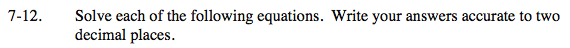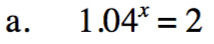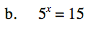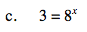Home > INT3 > Chapter 7 > Lesson 7.1.1 > Problem7-12

7-12.
1. Solve each of the following equations. Write your answers accurate to two decimal places. Homework Help ✎

1. 1.04x = 2

2. 5x = 15

3. 3 = 8xSince x is in the exponent in each equation, you will need to take the log of both sides.

$x \approx 17.673$$x \approx 1.68$